Courses

# Test: Speed, Time And Distance- 1

## 10 Questions MCQ Test IBPS PO Mains - Study Material, Online Tests, Previous Year | Test: Speed, Time And Distance- 1

Description
This mock test of Test: Speed, Time And Distance- 1 for Quant helps you for every Quant entrance exam. This contains 10 Multiple Choice Questions for Quant Test: Speed, Time And Distance- 1 (mcq) to study with solutions a complete question bank. The solved questions answers in this Test: Speed, Time And Distance- 1 quiz give you a good mix of easy questions and tough questions. Quant students definitely take this Test: Speed, Time And Distance- 1 exercise for a better result in the exam. You can find other Test: Speed, Time And Distance- 1 extra questions, long questions & short questions for Quant on EduRev as well by searching above.
QUESTION: 1

### A man covered a certain distance at some speed. If he had moved 3 kmph faster, he would have taken 40 minutes less. If he had moved 2 kmph slower, he would have taken 40 minutes more. What is the the distance in km?

Solution:

According to given condition

vt=(v+3)(t-2/3)

vt=(v-2)(t+2/3)

here v is velocity in kmph, t is time in hours and 2/3 is value of 40 min in hour on solving we will get t=10/3 and and v = 12 so distance = (10/3) * 12 = 40 kms

QUESTION: 2

### A man complete a journey in 10 hours. He travels first half of the journey at the rate of 21 km/hr and second half at the rate of 24 km/hr. Find the total journey in km.

Solution:

Let time taken to travel the first half = x hr

Then time taken to travel the second half = (10 - x) hr

Distance covered in the the first half = 21x [because, distance = time*speed]

Distance covered in the the second half = 24(10 - x)

Distance covered in the the first half = Distance covered in the the second half

So, 21x = 24(10 - x)

=> 45x = 240

=> x = 16/3

Total Distance = 2*21(16/3) = 224 Km [multiplied by 2 as 21x was distance of half way]

QUESTION: 3

### A and B walk around a circular track. A and B walk at a speed of 2 rounds per hour and 3 rounds per hour respectively. If they start at 8 a.m. from the same point in opposite directions, how many times shall they cross each other before 9.30 a.m.?

Solution:

Relative speed = Speed of A + Speed of B (∴ they walk in opposite directions) =2+3 = 5 rounds per hour

Therefore, they cross each other 5 times in 1 hour and 2 times in 1/2 hour

Time duration from 8 a.m. to 9.30 a.m. = 1.5 hour

Hence they cross each other 7 times before 9.30 a.m

QUESTION: 4

In covering a distance of 30 km, Arun takes 2 hours more than Anil. If Arun doubles his speed, then he would take 1 hour less than Anil. What is Arun's speed?

Solution:

If Arun doubles his speed, he needs 3 hour less. Double speed means half time. Hence, half of the time required by Arun to cover 30 km = 3 hour

i.e., Time required by Arun to cover 30 km = 6 hour

Arun's speed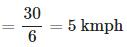QUESTION: 5

Excluding stoppages, the speed of a bus is 54 kmph and including stoppages, it is 45 kmph. For how many minutes does the bus stop per hour?

Solution:

Due to stoppages, it covers 9 km less.

Time taken to cover 9 km =(9/54 *60)min = 10 min.

QUESTION: 6

A car travels first 160 km at 64 km/hr and the next 160 km at 80 km/hr. What is the average speed for the first 320 km of the tour?

Solution: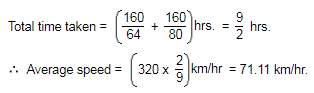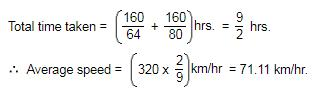QUESTION: 7

A car traveling with 5/7 of its actual speed covers 42 km in 1 hr 40 min 48 sec. What is the actual speed of the car?

Solution: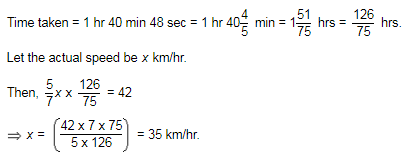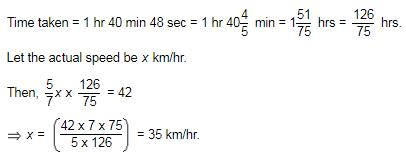QUESTION: 8

Two boys starts from the same place walking at the rate of 5 kmph and 5.5 kmph respectively in the same direction. What time will they take to be 8.5 km apart?

Solution:

The relative speed of the boys = 5.5kmph – 5kmph

= 0.5 kmph

Distance between them is 8.5 km

Time = Distance/Speed

Time= 8.5km / 0.5 kmph = 17 hrs

QUESTION: 9

Q. A man takes 5 hours 45 min. in walking to a certain place and riding back. He would have gained 2 hours by riding both ways. The time he would take to walk both ways, is:

Solution:

Given that time taken for riding both ways will be 2 hours lesser than the time needed for waking one way and riding back.

From this, we can understand that time needed for riding one way = time needed for waking one way - 2 hours

Given that time taken in walking one way and riding back = 5 hours 45 min

Hence The time he would take to walk both ways = 5 hours 45 min + 2 hours = 7 hours 45 min

QUESTION: 10

A person crosses a 600 m long street in 5 minutes. What is his speed in km per hour?

Solution:

Speed =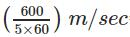= 2 m/sec = 2 x (18/5) km/hr = 7.2 km/hr